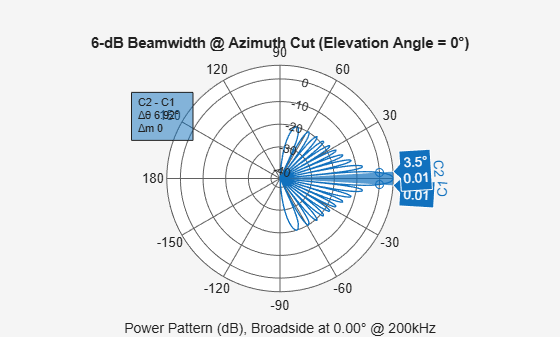# beamwidth

Compute and display beamwidth of an array

Since R2020b

## Syntax

``beamwidth(array,freq)``
``beamwidth(array,freq,Name,Value)``
``[bw,angles] = beamwidth(___)``

## Description

example

````beamwidth(array,freq)` plots the 2-D power pattern (in dB) of the `array` for all azimuth angles at a fixed elevation angle of zero degrees. The plot displays the half-power beamwidth (in degrees) at the frequency specified in `freq` (in Hz) and the angles (in degrees) in azimuth at which the magnitude of the power pattern decreases by 3 dB from the peak of the main beam.```
````beamwidth(array,freq,Name,Value)` plots the beamwidth with the specified parameter `Name` set to the specified `Value`. You can specify additional name-value pair arguments in any order as (`Name1,Value1,...,NameN,ValueN`).Example: `beamwidth(array,3e8,'Cut','Elevation')````

example

````[bw,angles] = beamwidth(___)` returns the angular beamwidth `bw` (in degrees). The function also returns the corresponding angle values (in degrees) that mark the beamwidth.```

## Examples

collapse all

Plot the beamwidth of a sonar array operating at a frequency of 2 kHz when the propagation speed of sound in water is 1500 m/s.

The sonar array consists of a 20-element uniform linear array (ULA). Consider the element of the ULA to be a backbaffled `phased.IsotropicProjector` with a `VoltageResponse` of 100 Volts and with a `FrequencyRange` from 10 Hz to 300 kHz. Create a `phased.ULA` object to model the uniform linear array.

``` projector = phased.IsotropicProjector('BackBaffled',true,... 'VoltageResponse',100,'FrequencyRange',[10 300000])```
```projector = phased.IsotropicProjector with properties: VoltageResponse: 100 FrequencyRange: [10 300000] BackBaffled: true ```
```myArray = phased.ULA('Element',projector,'NumElements',20,... 'ElementSpacing',1500/200e3/2)```
```myArray = phased.ULA with properties: Element: [1x1 phased.IsotropicProjector] NumElements: 20 ElementSpacing: 0.0037 ArrayAxis: 'y' Taper: 1 ```

Using the `beamwidth` function, calculate and plot the 6 dB beamwidth of the sonar array.

`beamwidth(myArray,200e3,'dBDown',6,'PropagationSpeed',1500)````ans = 6.9200 ```

Calculate the half-power beamwidth and angles of a 20-element uniform linear array (ULA) of cosine antenna elements.

Create a `phased.CosineAntennaElement` object with the `'CosinePower'` exponents set to 1.5.

`myAnt = phased.CosineAntennaElement`
```myAnt = phased.CosineAntennaElement with properties: FrequencyRange: [0 1.0000e+20] CosinePower: [1.5000 1.5000] ```

Create a `phased.ULA` object to model a 20-element ULA of cosine antenna elements. These elements are spaced at 0.5 meters on the azimuth plane.

`array = phased.ULA('Element',myAnt,'NumElements',20)`
```array = phased.ULA with properties: Element: [1x1 phased.CosineAntennaElement] NumElements: 20 ElementSpacing: 0.5000 ArrayAxis: 'y' Taper: 1 ```

Compute the beamwidth and angles of the array when it is operating at 3e8 Hz. Specify the beamwidth to be computed along the elevation plane.

`[BW,Ang] = beamwidth(array,3e8,'Cut','Elevation')`
```BW = 74.8200 ```
```Ang = 1×2 -37.4100 37.4100 ```

## Input Arguments

collapse all

Array of sensor elements, specified as one of the following System objects:

Frequency used to calculate the beamwidth, specified as a scalar in Hz.

Example: `3e8`

Data Types: `double`

### Name-Value Arguments

Specify optional pairs of arguments as `Name1=Value1,...,NameN=ValueN`, where `Name` is the argument name and `Value` is the corresponding value. Name-value arguments must appear after other arguments, but the order of the pairs does not matter.

Before R2021a, use commas to separate each name and value, and enclose `Name` in quotes.

Example: `beamwidth(array,3e8,'Cut','Azimuth','CutAngle',45)` plots the beamwidth of the array that is operating at a frequency of 0.3 GHz, with the slice direction set to `'Azimuth'`, and the cut angle set to 45 degrees.

The slice direction in azimuth-elevation space along which the beamwidth is computed, specified as the comma-separated pair consisting of `'Cut'` and `'Azimuth'` for the azimuth plane, and `'Cut'` and `'Elevation'` for the elevation plane.

Corresponding angle (in degrees) for the plane to get the required 2-D cut, specified as the comma-separated pair consisting of `'CutAngle'` and a scalar. If `'Cut'` is specified as `'Azimuth'`, then `'CutAngle'` (Elevation) should lie between [−90, 90] degrees. If `'Cut'` is specified as `'Elevation'`, then `'CutAngle'` (Azimuth) should lie between [−180, 180] degrees.

Data Types: `double`

Power value (in dB) from the peak of the main lobe, specified as the comma-separated pair consisting of `'dBDown'` and a positive scalar. The default value is 3 dB, which translates to half-power beamwidth. To calculate first-null beamwidth, specify the `'dBDown'` value as `Inf`.

Data Types: `single` | `double` | `int8` | `int16` | `int32` | `int64` | `uint8` | `uint16` | `uint32` | `uint64`

Propagation speed, specified as the comma-separated pair consisting of `'PropagationSpeed'` and a positive scalar (in m/s).

Data Types: `double`

Weights applied to the array of sensor elements, specified as the comma-separated pair consisting of `'Weights'` and a length-N column vector, where N is the number of elements in the array.

Data Types: `double`

## Output Arguments

collapse all

Angular beamwidth of the array of sensor elements, returned as a scalar in degrees.

Data Types: `double`

Angle values of the beamwidth, returned as a 1-by-2 vector. The two elements in the vector [amin, amax] define the beamwidth `bw` as amaxamin.

## Version History

Introduced in R2020b